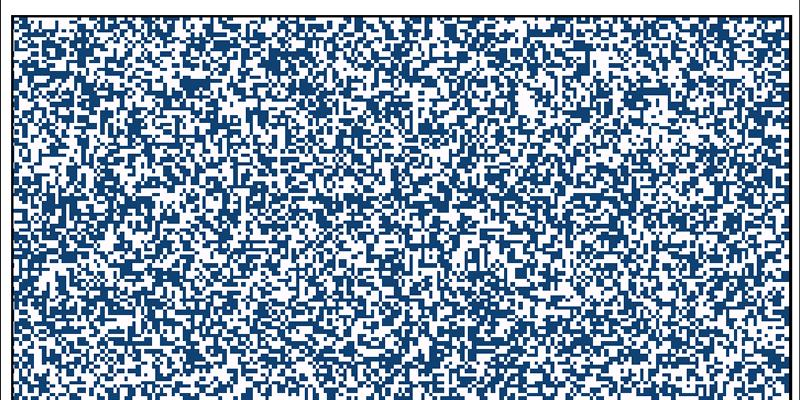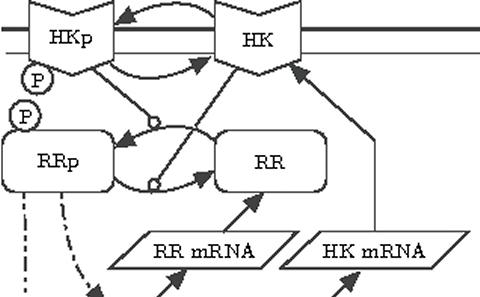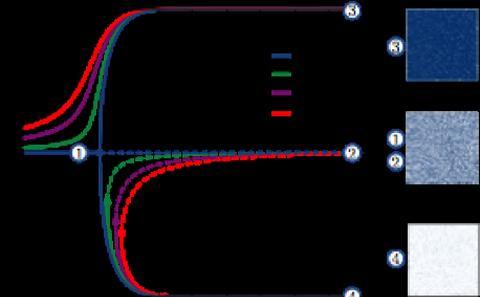The University of Southampton
Mathematical Sciences

# Research project: Stochastic Processes in Biology

Currently Active:
Yes

Traditionally modellers have treated biological processes as deterministic phenomena, that if repeated under exactly the same conditions would give exactly the same results. However, in recent decades, examples from different areas of biology such as gene regulation and cell division, as recently revealed by live cell images, have shown than many processes in cells and in molecular biology are inherently driven by stochastic (random) events.## Biochemical reactions

It is now well known that intrinsic noise can drive important biochemical processes. Intrinsic noise is associated with the uncertainty of knowing when a reaction occurs and what the reaction is. These effects are particularly accentuated when there are small numbers of molecules in the system. In this case the kinetics between the different species is best described by discrete-space continuous-time Markov processes. One of the main issues with this description is that even relatively small biological models very quickly become computationally intractable. Our main work in this area relates to the design and analysis of algorithms that are able to overcome this computational bottleneck.## Equation-free methods

Equation-free methods provide a bridge between microscopic descriptions of stochastic systems such as individual-based models, and macroscopic viewpoints of the same system, for example population-level perspectives. They not only allow for accelerated simulation at the macroscopic level but also enable system-level tasks, such as macroscopic bifurcation analysis. In the equation-free framework, we assume the existence of a closed macroscopic model in terms of a few state variables. However, instead of deriving an approximate macroscopic model analytically, we construct a computational superstructure, wrapped around a microscopic simulation. We have applied equation-free methods to understanding switching in two-component system signalling in bacteria, and have also developed improvements to the efficiency of the equation-free algorithm in the context of opinion dynamics.

### Related research groups

Applied Mathematics and Theoretical Physics
Mathematics in Biology and Medicine